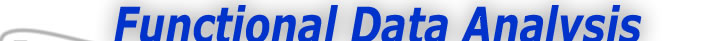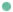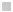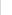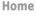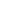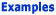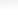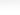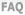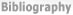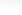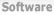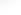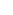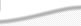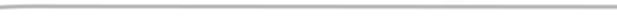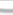BasisFunctions Goods Growth -What Data Am I working With -How do I define my B-spline basis? -What do we need to think about next? Weather
 From Raw Data to Functional Data Previous - 1 - 2 - 3 - Next Expertise: Beginner What do we need to think about next? Note that the curves seem to fit the height observations exactly. This process was simple, but is fitting the data exactly a good idea? We already acknowledged in the "Introduction to the Data" web page that the error in height measurements is of the order of three millimeters, so shouldn't we try to find curves that get closer to the truth rather than fitting the noisy data exactly? To see how to do this, go to the next sample analysis of these data where we consider smoothing. However, a simpler method to control the smoothness of a fit is to limit the number of basis functions. If knots are not specified, but the number of basis functions are, then knots are placed at equally spaced points. We know that about twelve basis functions are required to give a reasonable account of a growth curve. We could modify the basis creation command above to hgtbasis = create_bspline_basis([1,18], 12, 6); Previous - 1 - 2 - 3 - Next